1137字，阅读需时4分钟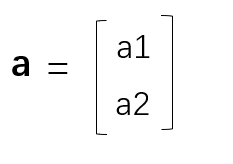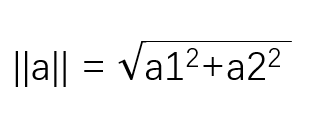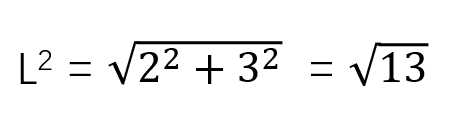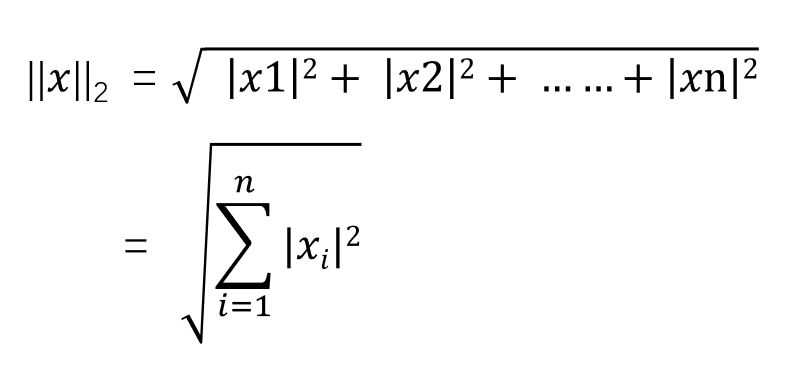L^2范数在机器学习中出现得十分频繁，经常简化表示为||x||，省略了下标2，下标2表示是L^2范数。L^1范数实际上是求向量中非零元素的绝对值之和。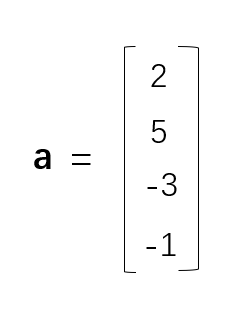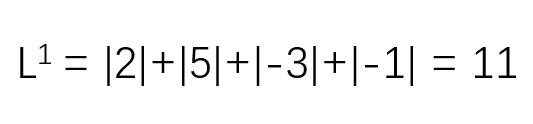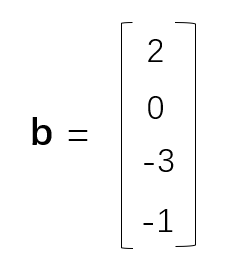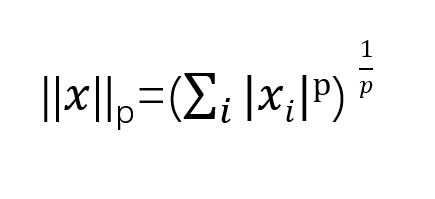```import numpy as np
#创建向量a
a =  np.array([2,-1,3,5,8])
if __name__ == '__main__':
#输出a的L2范数
print("a的L2范数为：%.2f" % (np.linalg.norm(a,2)))
#输出a的L1范数
print("a的L1范数为：%.2f" % (np.linalg.norm(a,1)))

#输出a的L0范数
print("a的L0范数为：%.2f" % (np.linalg.norm(a,0)))

#输出a的L∞范数
print("a的L∞范数为：%.2f" % (np.linalg.norm(a,np.inf)))```